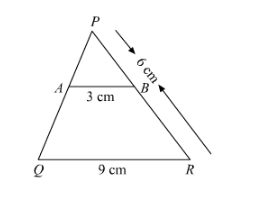# In the given figure, AB || QR. Find the length of PB.

Question:

In the given figure, AB || QR. Find the length of PB.Solution:

It is given that $A B \| Q R$

$A B=3 \mathrm{~cm}, Q R=9 \mathrm{~cm}$ and $P R=6 \mathrm{~cm}$

We have to find $P B$.

Since $\triangle P R Q \sim \triangle P A B$

$\Rightarrow \frac{A B}{Q R}=\frac{P B}{P R}$

So

$\frac{A B}{O R}=\frac{P B}{P R}$

$\frac{3 \mathrm{~cm}}{9 \mathrm{~cm}}=\frac{P B}{6 \mathrm{~cm}}$

$P B=2 \mathrm{~cm}$

Hence, $P B=2 \mathrm{~cm}$